# Quiz 9: Long-Lived Tangible and Intangible Assets

(1) Complete a depreciation schedule for each of the alternative methods: (a) Straight-line method:(b) Units-of-production depreciation method:(c) Double-declining-balance method:(2) Prepare the journal entry to account for the disposal of the asset: (a) Under Straight line depreciation: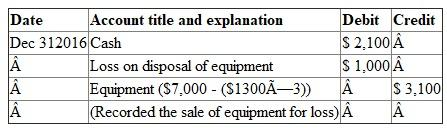(b) Units-of-production depreciation: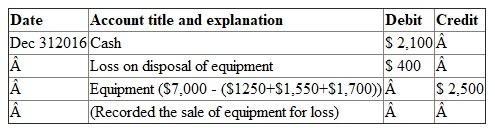(c) Double-declining-balance: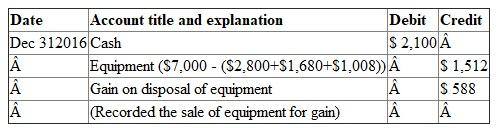(3) Create an income statement for the year ended December 31, 2016: (a) Under Straight line depreciation: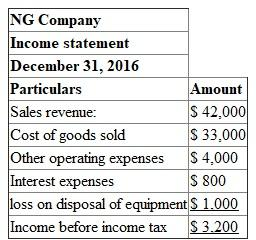(b) Units-of-production depreciation: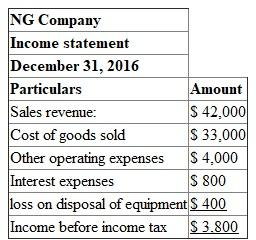(c) Double-declining-balance: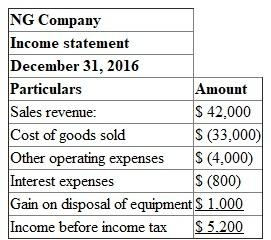(1) Prepare the journal entries to record the transactions and adjustments: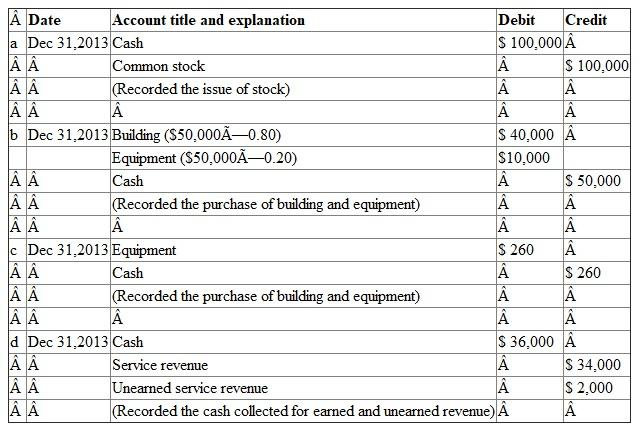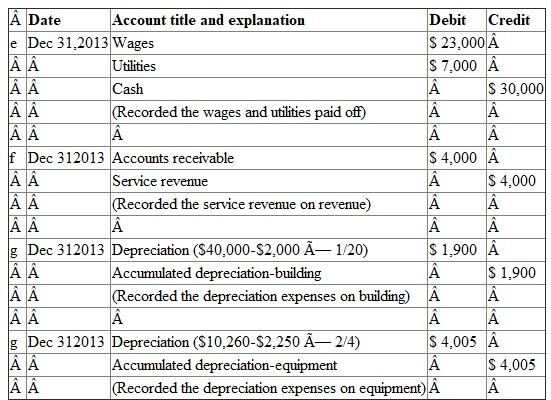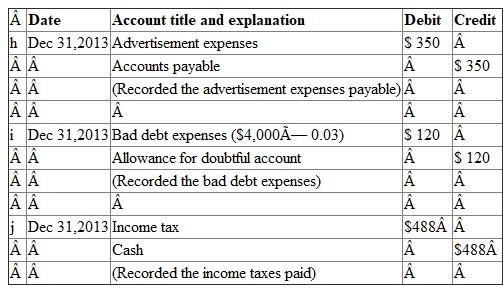(2) Prepare annual income statement, Statement of retained earnings, and classified balance sheet: Annual income statement: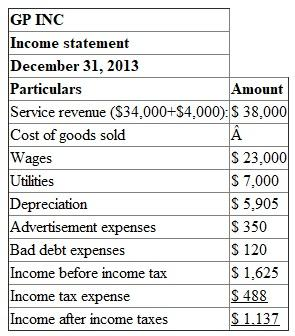Statement of retained earnings: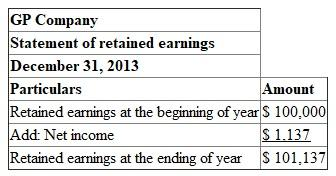Classified balance sheet: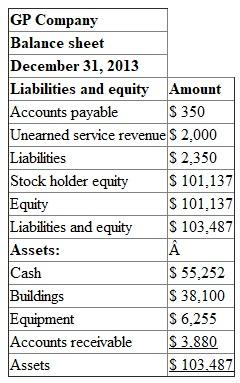(1) Compute the cost of each machine: The rational for capitalizing or expensing the various costs are based on one simple logic. The costs which are needed to prepare the asset for use must be capitalized and the rest must be expensed. Let us prepare the table with total costs of each machine as follows.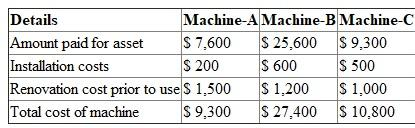(2) Give the journal entry to record depreciation expense at the end of year-1: Machine-A under Straight line depreciation method: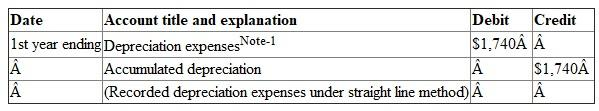Note-1:Machine-B under units-of-production depreciation method: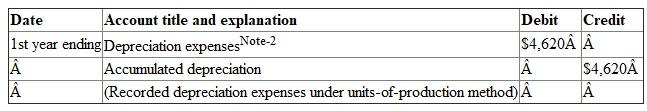Note-2:Machine-C under double-declining-balance method: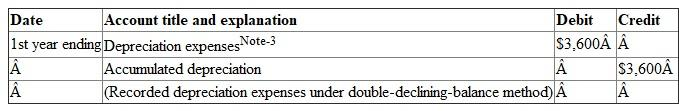Note-3: